# Voltage/current in circuit with two temperature sensors

Mårten

## Homework Statement

AD590 is a temperature sensor, through which a current of 1µA/K flows. AD590 is a sort of temperature sensitive transistor and can be interpreted as a current source. With two such sensors, you would like to measure the difference between temperatures T1 and T2 using a DMM. How to connect the sensors if you only have resistors of any kind and the two supply voltages + 12 V and - 12 V at your disposal? Draw a circuit, as simple as possible.

## Homework Equations

Ohm's law, Kirchhoff's circuit laws.

## The Attempt at a Solution

I came up with this circuit. The temperature sensors are the double ring symbols. Vcc=12V. I imagine that depending on the current I1 from e.g. the sensor in the left branch, the potential will fall 1k*I1 over the resistor to the left. The corresponding thing happens in the right branch, but with a different current I2. So now you should be able to read the temperature as the potential difference U. The temperature difference should be the the value for the potential difference measured in mV.
https://www.physicsforums.com/attachment.php?attachmentid=18447&stc=1&d=1239647527

But the correct answer is the following (temperature sensors are the circles with arrows inside):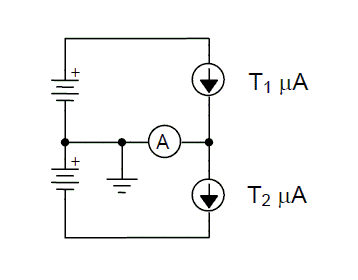Questions
1) Is my solution also correct? If not, why?

2) I understand that due to Kirchhoff's current law, you will have the difference in currents through the horizontal branch in the second drawing above. But a) why do I need two voltage sources (batteries)?, and b) why do I need a grounding point?, and c) is this grounding point the real planet earth, or is it just a reference potential? and finally d) if it's a reference potential, where is it really connected to the rest of the circuit? (I'm always getting confused when talking about grounding...)Any help appreciated!Last edited:

Staff Emeritus
Homework Helper
Hi Mårten,

Did you forget to post the schematic?

Mårten
Hi Redbelly,

Strange, it was there yesterday. I uploaded it via the manage attachment, and then used the img-tag... Maybe it was not the correct method. Anyway, here it is!P.s. Hm, it says Attachments Pending Approval down here... Maybe some admin took it away by mistake?

#### Attachments

Mårten
Hm, now my own suggestion circuit image disappeared as well. I'll try to upload it again. Don't know what I'm doing wrong when uploading these pics...https://www.physicsforums.com/attachment.php?attachmentid=18476&stc=1&d=1239746892

Staff Emeritus
Homework Helper
Okay, I'm seeing the "correct solution" circuit but not yours. When I go to quote your OP, I do see the link that is supposed to be your circuit:

https://www.physicsforums.com/attachment.php?attachmentid=18447&stc=1&d=1239647527

but trying to go there I get an error message, "Invalid Attachment specified".

You might try posting the image at www.photobucket.com instead (that's what I usually do). You can get a free account there.

That being said, do you at least understand how the "correct" circuit works? If the temperatures are equal, both current sources produce the same current, hence no current flows through the ammeter.

Mårten
Hi Redbelly again!(Or someone else.)

Sorry, for late reply to this thread. I'll take the whole matter from the beginning with - hopefully - working images (I used tinypic which I used before - both they and photobucket had some sort of 90 days limit for files anyway, so it doesn't matter). Here we go:

## Homework Statement

AD590 is a temperature sensor, through which a current of 1µA/K flows. AD590 is a sort of temperature sensitive transistor and can be interpreted as a current source. With two such sensors, you would like to measure the difference between temperatures T1 and T2 using a DMM. How to connect the sensors if you only have resistors of any kind and the two supply voltages + 12 V and - 12 V at your disposal? Draw a circuit, as simple as possible.

## Homework Equations

Ohm's law, Kirchhoff's circuit laws.

## The Attempt at a Solution

I came up with this circuit. The temperature sensors are the double ring symbols. Vcc=12V. I imagine that depending on the current I1 from e.g. the sensor in the left branch, the potential will fall 1k*I1 over the resistor to the left. The corresponding thing happens in the right branch, but with a different current I2. So now you should be able to read the temperature as the potential difference U. The temperature difference should be the the value for the potential difference measured in mV.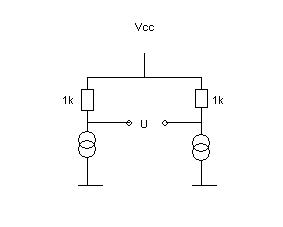But the correct answer is the following (temperature sensors are the circles with arrows inside):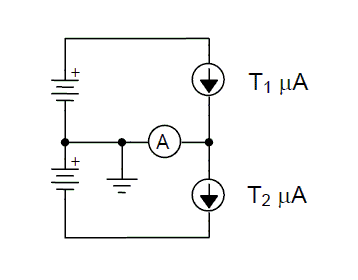Questions
1) Is my solution also correct? If not, why?

2) I understand that due to Kirchhoff's current law, you will have the difference in currents through the horizontal branch in the second drawing above. But a) why do I need two voltage sources (batteries)?, and b) why do I need a grounding point?, and c) is this grounding point the real planet earth, or is it just a reference potential? and finally d) if it's a reference potential, where is it really connected to the rest of the circuit? (I'm always getting confused when talking about grounding...)Any help appreciated!Staff Emeritus
Homework Helper
There is definitely more than 1 way to do this.

You're circuit will work in principle. A potential issue relates to accuracy. The 2 resistors in your circuit must be well matched so that zero ΔT results in zero signal (or reasonably close, it depends what are the requirements on accuracy).

Note that the "correct" circuit is a more direct measurement, the two currents are simply subtracted at the node common to the two AD590's to produce the ammeter current.

Yet another ΔT circuit is shown in Fig. 18, page 10 here:

Mårten
Ah, thanks!Then I was thinking, at least in principle, correct.

But now to the physics in the circuit with the ammeter. Do I really need two voltage sources? And what happens if I take away the grounding point? I don't know how to think there...

Staff Emeritus
Homework Helper
Yes, in that circuit separate voltage sources are needed for each AD590. Relative to the common point of the two AD590's, the other terminal is at a positive voltage on one of them, and at a negative voltage on the other.

If the grounding point is removed, the circuit will still theoretically work. If the voltages and currents are at safely low levels, it's not necessary to physically ground the circuit to the case. It would depend whether the device is powered, for example, by a wall plug (grounding necessary for safety reasons) or by two 9V batteries (grounding not necessary).

Hope that helps.

Mårten
Yes, in that circuit separate voltage sources are needed for each AD590. Relative to the common point of the two AD590's, the other terminal is at a positive voltage on one of them, and at a negative voltage on the other.
Hm... Still a little bit unsure. Suppose I take away the lower battery (I short-circuit there). Then, wouldn't I get a potential fall U1 first over the first sensor T1, and then an other potential fall U2 over the second sensor T2? Or do you mean that all the potential falls over T1, so there's nothing left to fall over T2, and why so in that case? See figure below how I mean: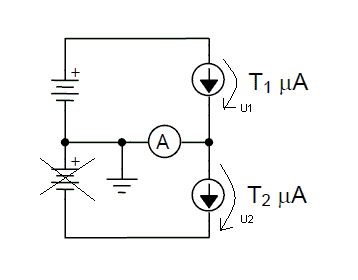Staff Emeritus
Homework Helper
Here's another view of the same circuit, which might help see what's going on better: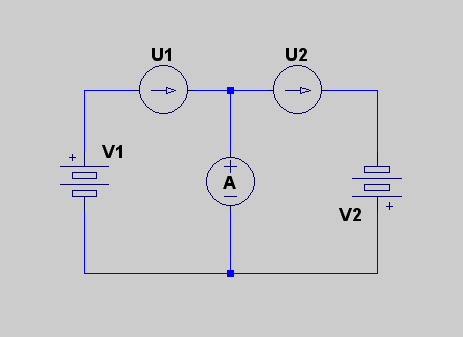Each voltage source supplies power to one and only one of the AD590's.

If we short out one of the voltage sources, say V2, the corresponding AD590 (U2) will not produce any appreciable current. If we consider the ammeter to be a short as well, that would mean zero voltage and zero current from U2. The ammeter will then simply register the current from U1.

Mårten
Okey, I think I got it sort of.

I guess I should know this... so if you have two branches and one of them have 0 resistance (the middle branch in the above fig), and the other have > 0 resistance (the branch to the right in the above fig, and suppose the battery to the right is not there as well), all the current will go in the branch with 0 resistance. Hm... but why is it really so? There's still a potential, why wont any electrons be affected and start to move in the branch to the right with > 0 resistance?

Last edited:
Staff Emeritus
Homework Helper
The branch with 0 resistance must have 0 voltage as well, because

V = I R = I*0 = 0​

The two branches are in parallel, and therefore have the same V=0.

The branch with R>0 must have zero current because

I = V/R = 0/R = 0 (only if R≠0)​

Mårten
Hm, interesting - thanks for that explanation, maybe pretty obvious...A final thing about grounding point then if you not have become weary of this thread...
If the grounding point is removed, the circuit will still theoretically work. If the voltages and currents are at safely low levels, it's not necessary to physically ground the circuit to the case. It would depend whether the device is powered, for example, by a wall plug (grounding necessary for safety reasons) or by two 9V batteries (grounding not necessary).
Am I right that in circuit schematics in general, there are two different kinds of grounding points? One of them is like the one you are describing, you ground it physically to the earth (i.e. the soil, planet earth). Another kind is just a reference point, normally equivalent to the minus terminal of the battery which you define as 0 V.

Is that correct?

Staff Emeritus
Homework Helper
There are those two uses, plus a third: the use of the chassis as "ground", though the chassis is often connected to the Earth anyway (through the ground wire in a 3-prong power plug).

Here is a more detailed explanation; scroll down to section IV for a diagram of the 3 types of ground:

http://www.ese.upenn.edu/rca/instruments/misctutorials/Ground/grd.html

The simple "reference point" type of ground is indicated by the triangle symbol, though I admit I have usually used the 3 horizontal lines without really thinking about this.

Mårten
Thanks a lot - that link was really helpful. I provide here a link to another thread I was part of last year, where grounding was discussed. Could be used for reference for anyone who pass by here:

And thanks again with all the help with the circuits in this thread!!